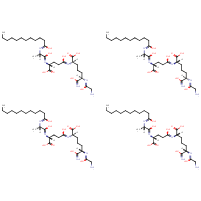Structure InformationCompound Identification

SMILES

CCCCCCCCCCCC(=O)N[C@@H](C)C(=O)N[C@H](CCC(=O)N[C@H](CCC[C@@H](NC(=O)CN)C(N)=O)C(O)=O)C(O)=O.CCCCCCCCCCCC(=O)N[C@@H](C)C(=O)N[C@H](CCC(=O)N[C@H](CCC[C@H](NC(=O)CN)C(N)=O)C(O)=O)C(O)=O.CCCCCCCCCCCC(=O)N[C@@H](C)C(=O)N[C@H](CCC(=O)N[C@@H](CCC[C@@H](NC(=O)CN)C(N)=O)C(O)=O)C(O)=O.CCCCCCCCCCCC(=O)N[C@@H](C)C(=O)N[C@H](CCC(=O)N[C@@H](CCC[C@H](NC(=O)CN)C(N)=O)C(O)=O)C(O)=O

InChIKey

InChIKey=QMVQDCBTYJXPRK-OFGBJLENSA-N

Formula

C116H208N24O36

Mass

2515.072

### Export to:

Taxonomic Classification

Taxonomy Tree

Kingdom

Organic compounds

Superclass

Organic acids and derivatives

Class

Carboxylic acids and derivatives

Subclass

Amino acids, peptides, and analogues

Intermediate Tree Nodes

Peptides

Direct Parent

Dipeptides

Alternative Parents

Molecular Framework

Not available

Substituents

Alpha-dipeptide - Gamma-glutamyl alpha-amino acid - Glutamine or derivatives - N-acyl-alpha amino acid or derivatives - N-acyl-alpha-amino acid - N-acyl-l-alpha-amino acid - Alpha-amino acid amide - Alanine or derivatives - N-substituted-alpha-amino acid - Alpha-amino acid or derivatives - Medium-chain fatty acid - Amino fatty acid - Dicarboxylic acid or derivatives - N-acyl-amine - Fatty acyl - Fatty acid - Fatty amide - Amino acid or derivatives - Amino acid - Carboxamide group - Secondary carboxylic acid amide - Primary carboxylic acid amide - Carboxylic acid - Organic oxide - Hydrocarbon derivative - Organic oxygen compound - Carbonyl group - Organic nitrogen compound - Amine - Primary aliphatic amine - Primary amine - Organooxygen compound - Organonitrogen compound - Aliphatic acyclic compound

Description

This compound belongs to the class of organic compounds known as dipeptides. These are organic compounds containing a sequence of exactly two alpha-amino acids joined by a peptide bond.

External Descriptors

Not available